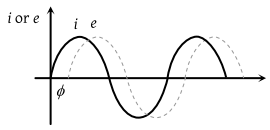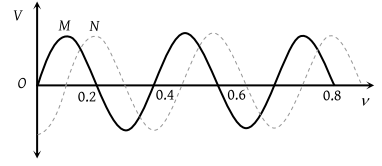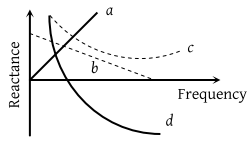When an ac source of e.m.f. $e={E}_{0}\mathrm{sin}\left(100\text{\hspace{0.17em}}t\right)$ is connected across a circuit, the phase difference between the e.m.f. e and the current i in the circuit is observed to be &pi:/4, as shown in the diagram. If the circuit consists possibly only of RC or LC in series, find the relationship between the two elements(1) $R=1k\Omega ,\text{\hspace{0.17em}}C=10\mu F$

(2) $R=1k\Omega ,\text{\hspace{0.17em}}C=1\mu F$

(3) $R=1k\Omega ,\text{\hspace{0.17em}}L=10H$

(4) $R=1k\Omega ,\text{\hspace{0.17em}}L=1H$

Concept Questions :-

Different types of AC Circuits
High Yielding Test Series + Question Bank - NEET 2020

Difficulty Level:

Two sinusoidal voltages of the same frequency are shown in the diagram. What is the frequency, and the phase relationship between the voltagesFrequency in Hz Phase lead of N over M in radians (1) 0.4 –π/4 (2) 2.5 –π/2 (3) 2.5 +π/2 (4) 2.5 –π/4

Concept Questions :-

AC vs DC
High Yielding Test Series + Question Bank - NEET 2020

Difficulty Level:

Which of the following plots may represent the reactance of a series LC combination(1) a

(2) b

(3) c

(4) d

Concept Questions :-

Different types of AC Circuits
High Yielding Test Series + Question Bank - NEET 2020

Difficulty Level:

A filament bulb (500 W,100 V) is to be used in a 230 V main supply. When a resistance R is connected in series, it works perfectly and the bulb consumes 500 W. The value of R is

(a) 230$\Omega$          (b) 46$\Omega$

(c) 26$\Omega$            (d) 13$\Omega$

High Yielding Test Series + Question Bank - NEET 2020

Difficulty Level:

Which of the following combinations should be selected for better tuning of an L-C-R circuit used for communication ?

(a)

(b)

(c)

(d)

High Yielding Test Series + Question Bank - NEET 2020

Difficulty Level:

The potential differences across the resistance, capacitance and inductance are 80V, 40Vand 100V respectively in an L-C-R circuit. The power factor of this circuit is

(a) 0.4              (b) 0.5

(c) 0.8              (d) 1.0

High Yielding Test Series + Question Bank - NEET 2020

Difficulty Level:

A 100 $\Omega$ resistance and a capacitor of 100 $\Omega$ reactance are connected in series across a 220 V source. When the capacitor is 50% charged, the peak value of the displacement current is

(a) 2.2 A              (b) 11A

(c) 4.4A               (d) 11$\sqrt{2}$A

Concept Questions :-

Different types of AC Circuits
High Yielding Test Series + Question Bank - NEET 2020

Difficulty Level:

A small-signal voltage V(t)=Vo sinωt is applied across an ideal capacitor C
(a) over a full cycle, the capacitor C does not consume any energy from the voltage         source
(b) current I(t) is in phase with voltage V(t)
(c) current I(t) leads voltage V(t) by 180°
(d) current I(t) lags voltage V(t) by 90°

Concept Questions :-

Rms and average values
High Yielding Test Series + Question Bank - NEET 2020

Difficulty Level:

An inductor 20 mH, a capacitor 50μF, and a resistor 40Ω are connected in series across a source of emf V=10sin340t. The power loss in the AC circuit is:

1. 0.67 W

2. 0.78W

3. 0.89 W

4. 0.46 W

Concept Questions :-

Different types of AC Circuits
High Yielding Test Series + Question Bank - NEET 2020

Difficulty Level:

A resistance 'R' draws power 'P' when connected to an AC source. If an inductance is now placed in series with the resistance, such that the impedance of the circuit becomes 'Z' the power drawn will be:

Concept Questions :-

Different types of AC Circuits